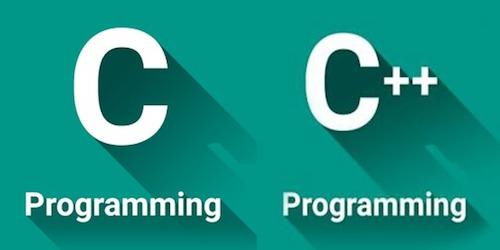# How To Round Numbers In C And C++

Rounding numbers is not a difficult task. The truth is that we keep doing it every day and most of the time, we don’t even realize it. No matter if you are splitting the bill with your colleagues or at the grocery store, you just round numbers naturally

However, it’s important to consider that some jobs require the use of different languages or software to round numbers. These may include using Microsoft Excel, java, python, javascript, C, and C++. So, today, we decided to show you how to round numbers in C and C++.

## How To Round Numbers In C And C++

Today, we decided to start by showing you a simple example. Let’s say that you want to round off a floating point value to two places. For example, 5.567 should become 5.57, and 5.534 should become 5.53.

One of the most important things you need to keep in mind is that there are different methods you can use.

Discover the best tips and tricks to round a number in Excel.

## Method #1: Using Float Precision:

In this case, the code is as follows:

#include <iostream>

using namespace std;

int main()

float var = 37.66666;

// Directly print the number with .2f precision

printf(“%.2f”, var);

return 0;

In this case, you will get an output of 37.67.

Learn how to round numbers in Python.

## Method #2: Using Integer Typecast:

If you are in Function, this is how to return two decimal point value:

#include <iostream>

using namespace std;

float round(float var)

// 37.66666 * 100 =3766.66

// 3766.66 + .5 =3767.16 for rounding off value

// then type cast to int so value is 3767

// then divided by 100 so the value converted into 37.67

float value = (int)(var * 100 + .5);

return (float)value / 100;

int main()

float var = 37.66666;

cout << round(var);

return 0;

In this case, you will get an output of 37.67.

## Method #3: Using Sprintf() And Sscanf():

In this case, the code is as follows:

#include <iostream>

using namespace std;

float round(float var)

// we use array of chars to store number

// as a string.

char str;

// Print in string the value of var

// with two decimal point

sprintf(str, “%.2f”, var);

// scan string value in var

sscanf(str, “%f”, &var);

return var;

int main()

float var = 37.66666;

cout << round(var);

return 0;

In this case, you will get an output of 37.67.

## C – Round() Function

When you want to use the round() function in C, it’s important to consider some factors:

• The round( ) function in C returns the nearest integer value of the float/double/long double argument passed to this function.
• If the decimal value is from ”.1 to .5″, it returns an integer value less than the argument. If the decimal value is from “.6 to .9″, it returns the integer value greater than the argument.
• The ”math.h” header file supports round() function in C language. Syntax for round( ) function in C is as follows:

double round (double a);

float roundf (float a);

long double roundl (long double a);

## C – Round() Function – Simple Example

#include <stdio.h>

#include <math.h>

int main()

{

float i=5.4, j=5.6;

printf(“round of %f is %f\n”, i, round(i));

printf(“round of %f is %f\n”, j, round(j));

return 0;

}

In this case, you get the following output:

• round of 5.400000 is 5.000000
• round of 5.600000 is 6.000000